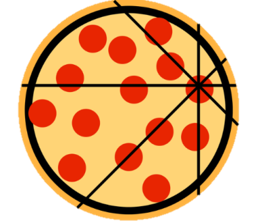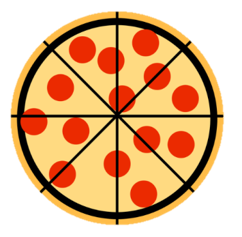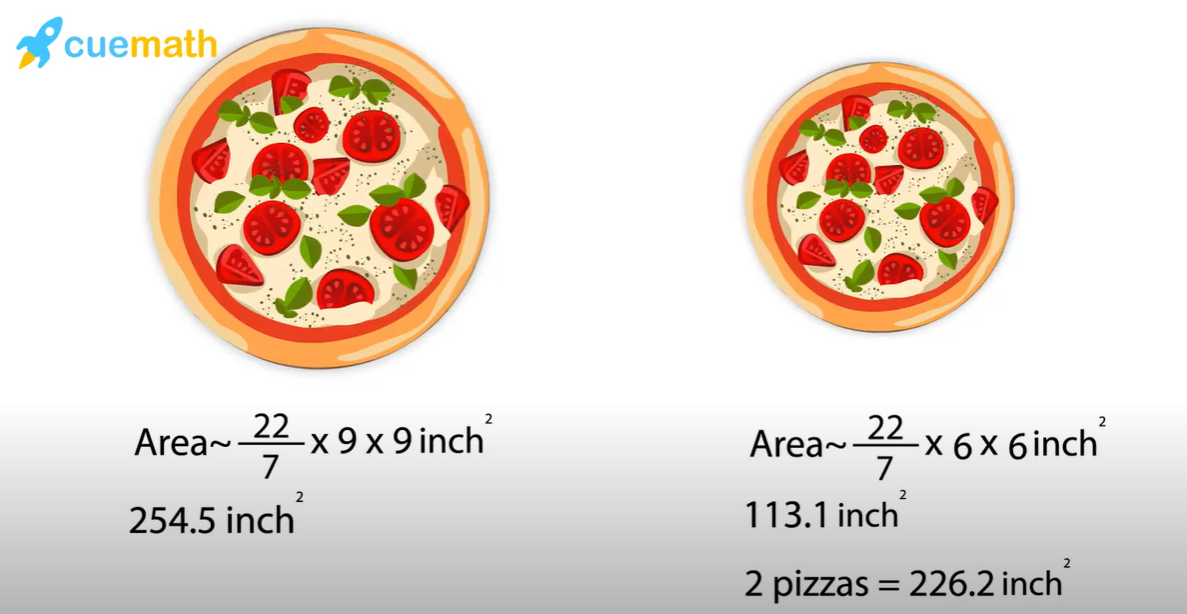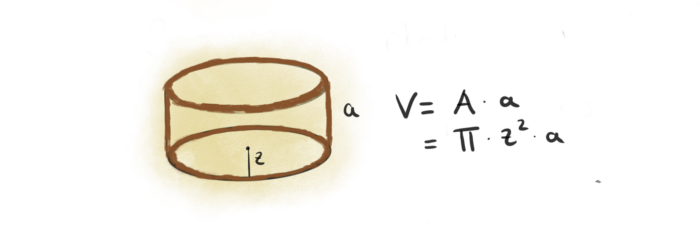Math Puzzles

# How can you solve the veg pizza dispute?

1.1k views

 1 Introduction 2 Math and Pizza relationship: The Pizza Theorem 3 Volume of Pizza 4 Conclusion 5 About Cuemath 6 Frequently Asked Questions (FAQs) 7 External References

## Introduction

Do you know what happens when a team of math geeks comes together to have a debate over warm slices of veg pizzas?

It becomes a session of finding math in food.

Well, that’s exactly what happened at the Cuemath HQ today. Upon ordering a pizza that apparently came in a square box, we decided to wrack our brains with the most challenging math question we could come up with – by looking at a pizza.### How can you solve the veg pizza dispute-PDF

Everyone Love Pizzas! In this blog, we discuss how to solve the veg pizza dispute through the pizza theorem and pizza problems. A beautiful relationship is built around math and pizza called The Pizza Theorem. It is named after a traditional pizza slicing technique and states the equality of two areas when a person divides a pizza from a particular point. Here is a downloadable PDF to explore more.

## Math and Pizza relationship: The Pizza Theorem

Math is so interesting that a circle shape pizza is delivered in a square pizza box and therefore sliced into triangular slices.

A beautiful relationship is built around math and pizza called The Pizza Theorem. It is named after a traditional pizza slicing technique and states the equality of two areas when a person divides a pizza from a particular point.

Example: Ramesh and Suresh are best friends and they decided to have a study session together. Both of them are determined to make it into Maths Olympiad Final, hence they decided to study together. They ordered pizza and Suresh volunteered to cut the Pizza into slices. Ramesh gave him the following instructions to cut the pizza:

• Cut it into 8 pieces so it can be divided equally among us.

• Make 4 slices in the center.

• The cut of the pizza will be in Horizontal and vertical lines.

• Two more cuts at 45-degree angles.Suresh enters the kitchen but gets back with the pizza cut in the following way.## Do you think that both of them will get an equal share of the pizza?

Yes, they will. This is the manifestation of the pizza theorem. According to it, if the pizza is divided into two semi circle, 4, 8, 12, 16.....sections by making cuts at equal angles from a particular point, then the sum of the areas of alternate pieces will be equal.

So, now whenever you go out to have a pizza, keep this pizza theorem in mind and ask the pizza person not to cut the pizza for you. Instead, ask a cutter from him and let your inner mathematician play some magic on the pizza.

## Volume of Pizza

How much pizza is in a pizza?

Let's calculate the Area of two pizzas, a large pizza is 18 inches in diameter and regular pizza is 12 inches.

Area of the circle  $$=\pi r^2$$

Since we already know the diameter of the pizzas. The radius of the pizza will be r = diameter/2, which is 9 inches for the large pizza and 6 inches for the regular pizzas.From the above pizza image, the Area of 18 inch pizza is 254.5 square inches and the area of 9 inch pizza is 113.1 square inches. So, the area of two regular pizzas is 226.2 square inches that is less than area of a large pizza.

So always go for a large instead of two regular pizzas.

To know other facts about pizza, do check out our blog: Are you paying extra for your pizza?

Let the pizza now have a height of 'a' and let’s calculate the volume!If z = radius of pizza.

a = height of the pizza.

Then the volume of the pizza, considering it as a cylinder =$$pi\times z\times z\times a$$ = pizza

The Volume of Pizza with height 'a' and radius 'z' is Pizza.

## Conclusion

Math skills are fundamental in every field of life. Also, it helps us get some extra pizza, and who wouldn't love some more pizza?

Everyone loves pizza and kids even more so. Can we relate this unique mouth-watering dish to a mathematical logic?  Think of other creative ways to make learning Math fun.

Cuemath, a student-friendly mathematics platform, conducts regular Online Live Classes for academics and skill-development and their Mental Math App, on both iOS and Android, is a one-stop solution for kids to develop multiple skills.

## How many slices in medium pizza?

There can be a range of 4-8 of 8 slices in a medium-size pizza.

## How is pizza related to math?

Pizza can be related to math in many ways such as Shapes, area, volume. The most beautiful relationship between pizza and math is the Pizza theorem.

## How big is 11 inch pizza?

11 inch pizza is a large pizza that can be served to 3-4 people. Area of 11 inch pizza is 95.03 square inches.